﻿ 基于卷积神经网络和多类SVM的交通标志识别
«上一篇文章快速检索 高级检索

 应用科技2018, Vol. 45Issue (5): 71-76, 81  DOI: 10.11991/yykj.2017100090

### 引用本文SONG Jiarong, YANG Zhong, ZHANG Tianyi, et al. Traffic signs recogniton based on convolutional neural networks and multi-class SVM[J]. Applied Science and Technology, 2018, 45(5), 71-76, 81. DOI: 10.11991/yykj.201710009.### 文章历史

Traffic signs recogniton based on convolutional neural networks and multi-class SVM
SONG Jiarong, YANG Zhong, ZHANG Tianyi, HAN Jiaming, ZHU Jiayuan
College of Automation Engineering, Nanjing University of Aeronautics and Astronautics, Nanjing 211106, China
Abstract: In order to realize recognition of traffic signs with higher accuracy in complex environment and to acquire a classifying network that works well with small samples, a traffic signs recognition system based on the convolutional neural networks (CNNs) and multi-class support vector machine (SVM) is proposed in this paper. It does not need to design artificial feature extraction algorithm and a classification model with higher accuracy can be trained on a small sample training set. Besides, the training learning strategy is used to avoid reinitializing CNNs, so it avoids overfitting while saving a large amount of samples and time efficiently. Experimental results demonstrate that the classification model obtained in the small sample training set has good performance and has reliable recognition ability and good classification results for samples even in the condition of complex background and severe distortion.
Keywords: traffic signs recognition    CNNs    deep learning    transfer learning    multi-class SVM    overfitting    softmax    AlexNet

1 卷积神经网络和SVM 1.1 AlexNet网络

AlexNet网络是著名的卷积分类网络，可成功实现对1 000类别物体的分类。其结构可以分为特征提取器和分类器2部分。

 $p({y^{(i)}} = j|{x^{(i)}};{\theta }) = \frac{{{{\rm e}^{{\theta }_i^{\rm{T}}{{x}^{(i)}}}}}}{{\sum\nolimits_{l = 1}^k {{{\rm e}^{{\theta }_l^{\rm{T}}{{x}^{(i)}}}}} }}$ (1)

1.2 标准SVM

SVM是基于结构风险最小化理论的有监督学习算法，通过寻找最优分割超平面来实现分类。相比于神经网络，SVM在小样本数据集上也能表现出良好的分类效果，因而得到广泛使用。标准的SVM通过寻求式（2）的最优解来找到最优超分割平面。

 $\left\{ \begin{array}{l}{\min _{{w},b}}\frac{1}{2}||{w}|{|_2}\\{y^{(i)}}({w} \cdot {{x}^{(i)}} + b) \geqslant 1(i = 1,2, \cdots ,m)\end{array} \right.$ (2)

2 分类模型设计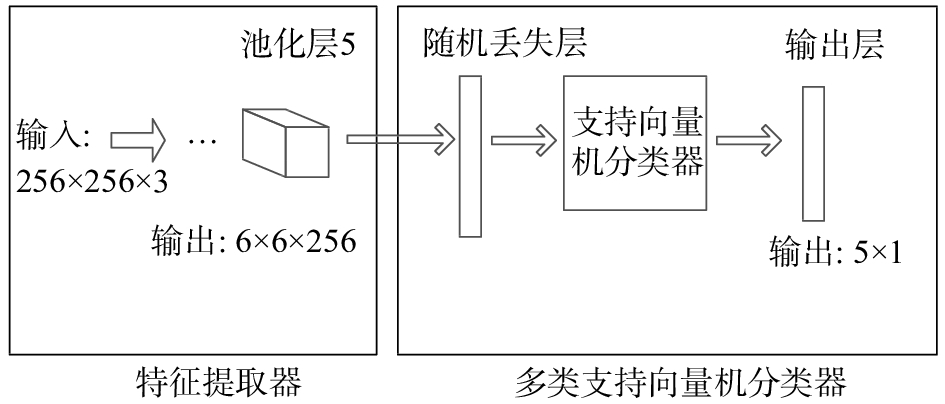Download: 图 1 模型结构

2.1 特征提取器

2.2 分类器

 ${L_i} = \sum\limits_{j \ne {y_i}} {\max (0,{s_j} - {s_i} + 1)}$
 $L = \sum\limits_{i = 1}^m {{L_i}}$ (3)

 $L = \sum\limits_{i = 1}^m {{L_i}} + \lambda ||{w}|{|_2}$

2.3 测试阶段对dropout层的处理

 ${i_{{\rm{withdropout}}}} = p\sum {{w_i}{x_i}} + b$ (4)
 ${i_{{\rm{withoutdropout}}}} = \sum {{w_i}{x_i}} + b$ (5)

3 实验结果与分析 3.1 实验数据表 1 实验样本组成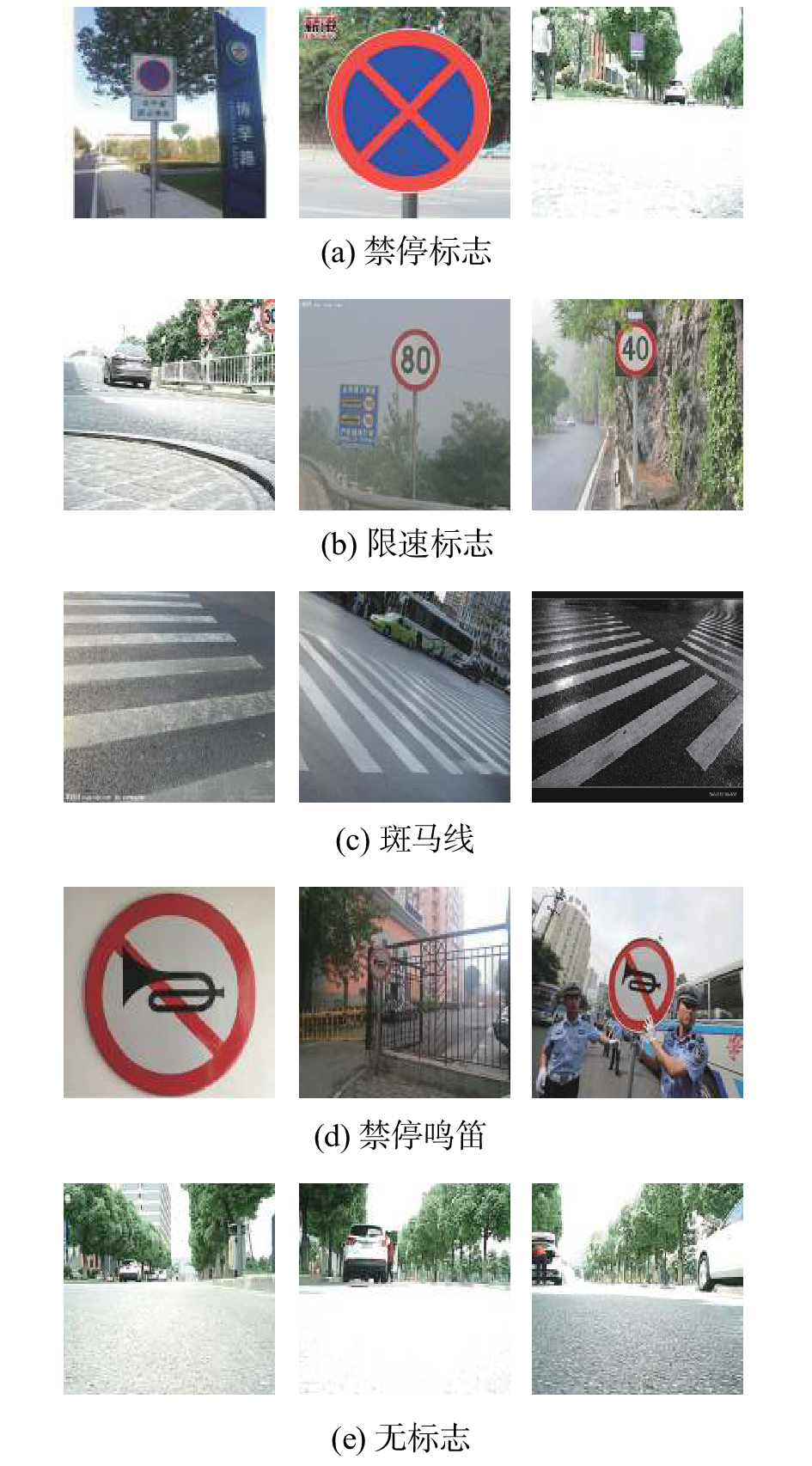Download: 图 2 部分训练样本展示
3.2 分类模型的训练与测试 3.2.1 小样本数据集上SVM与softmax对比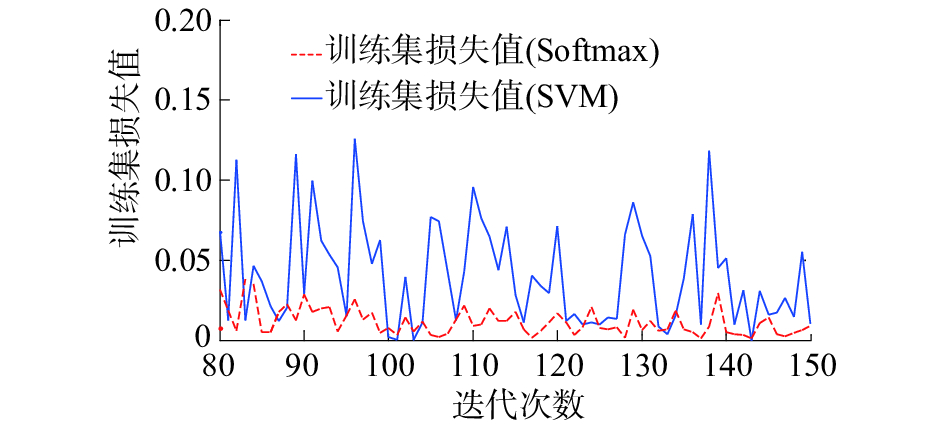Download: 图 3 Softmax与SVM训练损失值对比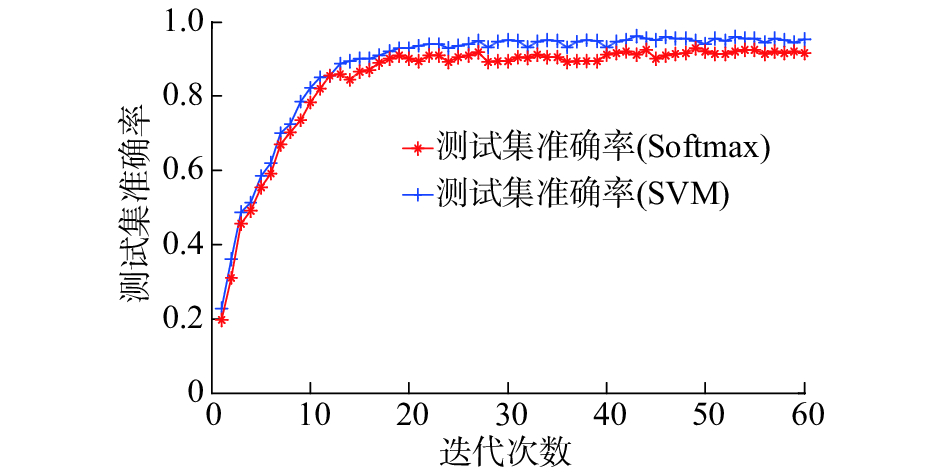Download: 图 4 Softmax与SVM测试准确率对比表 2 Softmax与SVM实验结果统计

3.2.2 dropout层的影响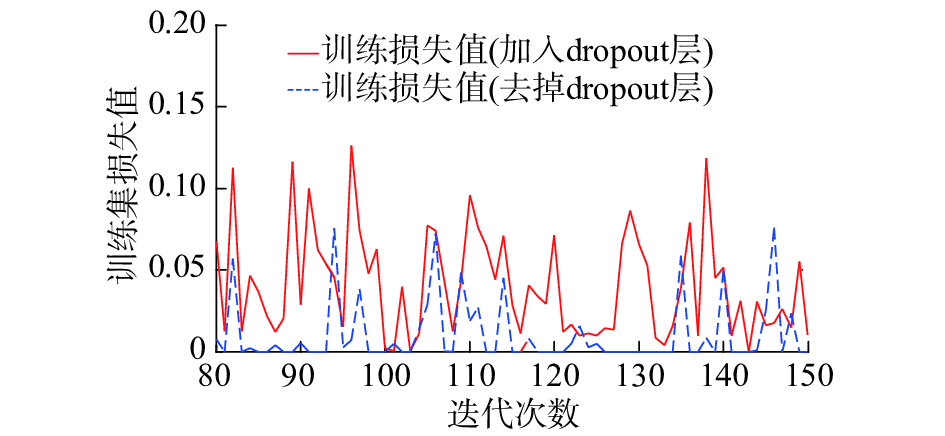Download: 图 5 有无dropout层训练损失值对比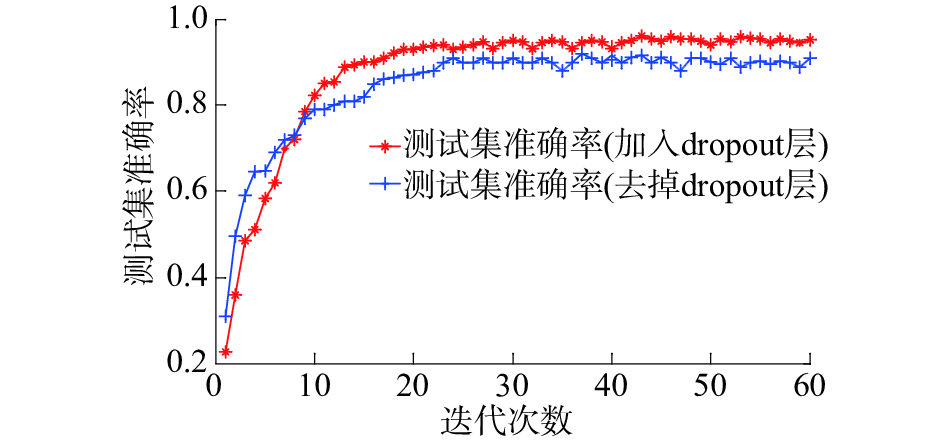Download: 图 6 有无dropout层测试准确率对比表 3 有无dropout层实验结果统计

3.3 分类模型的实物预测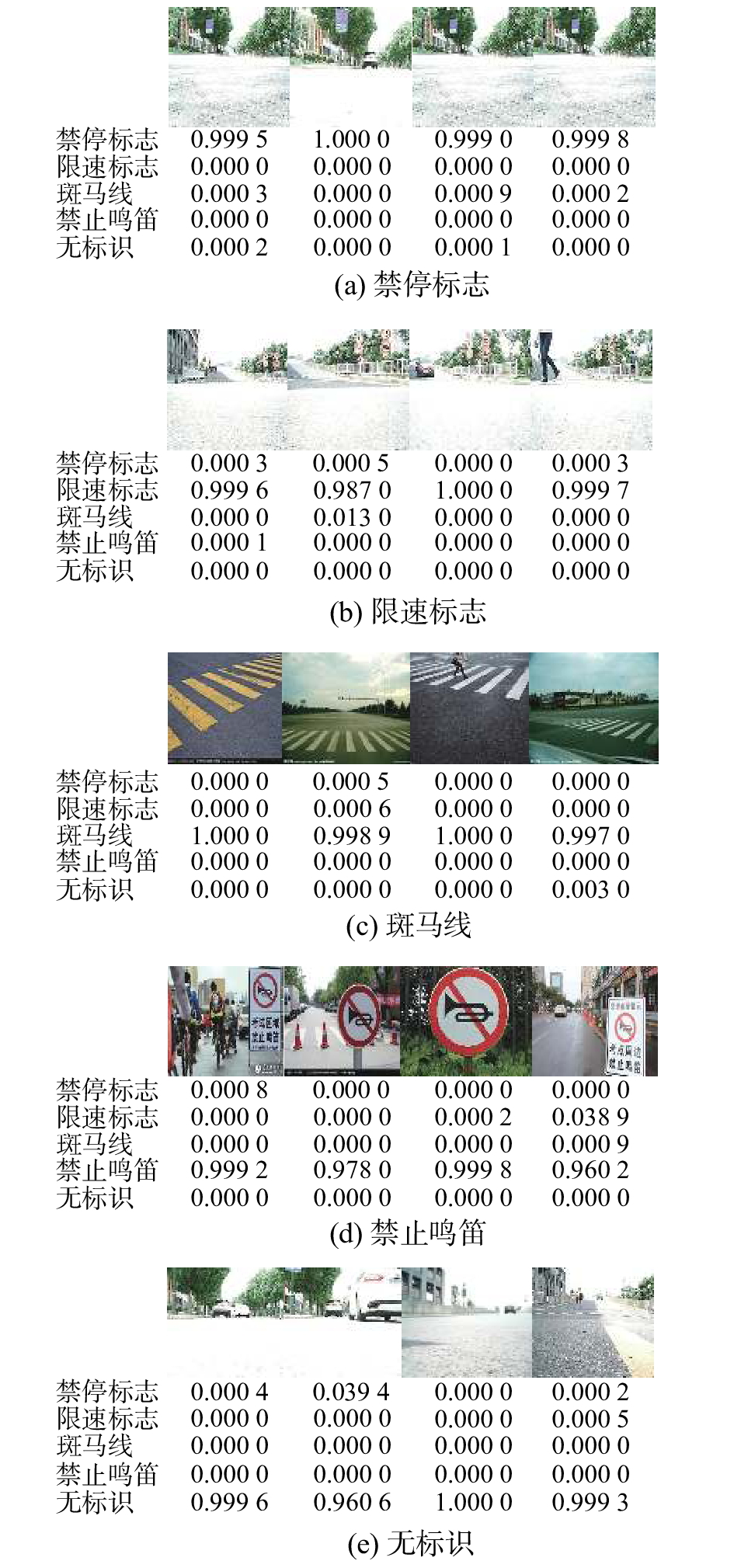Download: 图 7 实物预测结果

4 结论

1）在小样本数据集条件下，SVM分类器相比于softmax分类器有更好的分类结果。

2）本文利用卷积神经网络与多类SVM分类器构建了对交通标志的识别模型。同时为了防止过拟合现象的发生，在多类SVM分类器中融入了dropout层。并通过对于实验证明了加入dropout层的模型有更好的分类准确度。

3) 分类模型的实物预测实验证明该模型对环境变化有较强的鲁棒性。

  王方石, 王坚, 李兵, 等. 基于深度属性学习的交通标志检测[J]. 吉林大学学报: 工学版, 2018, 48(1): 319-329. (0)  吕耀坤. 基于卷积神经网络的实景交通标志识别[J]. 物联网技术, 2017, 7(1): 29-30. (0)  黄琳, 张尤赛. 应用深层卷积神经网络的交通标志识别[J]. 现代电子技术, 2015, 38(13): 101-106. DOI:10.3969/j.issn.1004-373X.2015.13.028 (0)  郭显娥, 武伟, 刘春贵, 等. 多类SVM分类算法的研究[J]. 山西大同大学学报: 自然科学版, 2010, 26(3): 6-8, 14. (0)  TAJBAKHSH N, SHIN J Y, GURUDU S R, et al. Convolutional neural networks for medical image analysis: full training or fine tuning[J]. IEEE transactions on medical imaging, 2016, 35(5): 1299-1312. DOI:10.1109/TMI.2016.2535302 (0)  MARMANIS D, DATCU M, ESCH T, et al. Deep learning earth observation classification using ImageNet Pretrained networks[J]. IEEE geoscience and remote sensing letters, 2016, 13(1): 105-109. DOI:10.1109/LGRS.2015.2499239 (0)  KRIZHEVSKY A, SUTSKEVER I, HINTON G E. ImageNet classification with deep convolutional neural networks[C]//Proceedings of the 25th International Conference on Neural Information Processing Systems. Lake Tahoe, Nevada, 2012: 1097-1105. (0)  GU Yingxin, WYLIE B K, BOYTE S P, et al. An optimal sample data usage strategy to minimize overfitting and underfitting effects in regression tree models based on remotely-sensed data[J]. Remote sensing, 2016, 8(11): 943. DOI:10.3390/rs8110943 (0)  SRIVASTAVA N, HINTON G, KRIZHEVSKY A, et al. Dropout: a simple way to prevent neural networks from overfitting[J]. Journal of machine learning research, 2014, 15(1): 1929-1958. (0)  张冬生. 支持向量机在分类问题中的应用研究[J]. 黑龙江科技信息, 2010(35): 64. DOI:10.3969/j.issn.1673-1328.2010.35.059 (0)  WU Yichao, LIU Yufeng. Robust truncated hinge loss support vector machines[J]. Journal of the American statistical association, 2007, 102(479): 974-983. DOI:10.1198/016214507000000617 (0)  刘建伟, 李双成, 罗雄麟. p范数正则化支持向量机分类算法 [J]. 自动化学报, 2012, 38(1): 76-87. (0)